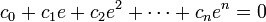# multi nested loop, any hints?

multi nested loop, any hints?

I need to calculate the roots of. In this case, multi-nested loop (>4) is need. How to let GPU calculate it with the device code parallely and efficently?

thanks

currently I have a stupid solution: make and fill a array c in host code first, then this array is used as a 2-d array

for example, for 4-order equation

``````//this is code totally on cpu

for(i=0;i<I;i++)

for(j=0;j<J;j++)

for(k=0;k<K;k++)

for(l=0;l<L;l++)

for(m=0;m<M;m++)

fillRootArray(getroot(i,j,k,l,m));
``````
``````//this code use cpu and gpu

//but I have to fill the array by CPU code

//and the "some idx" is hard to write if I get more dimension

__host__ fillarray()

{

for(i=0;i<I;i++)

for(j=0;j<J;j++)

for(k=0;k<K;k++)

for(l=0;l<L;l++)

for(m=0;m<M;m++)

c[some idx]=[i,j,k,l,m];

// as a result c=[[0,0,0,0,0];[0,0,0,0,1];.....;[I-1, J-1, K-1, L-1, M-1]];

}

__device__ getroot()

{

fillRootArray(getroot(c));

}
``````

In the case fillRootArray(getroot(i,j,k,l,m)); does not depend on i-1 or j-1 or k-1 or l-1 or m-1 you can just create a grid in which you have 5 independent indices obtained from the threadIdx.x and blockIdx.x variables.